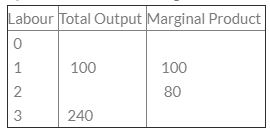Courses

# Test: Theory Of Production- 2

## 30 Questions MCQ Test Business Economics for CA Foundation | Test: Theory Of Production- 2

Description
This mock test of Test: Theory Of Production- 2 for CA Foundation helps you for every CA Foundation entrance exam. This contains 30 Multiple Choice Questions for CA Foundation Test: Theory Of Production- 2 (mcq) to study with solutions a complete question bank. The solved questions answers in this Test: Theory Of Production- 2 quiz give you a good mix of easy questions and tough questions. CA Foundation students definitely take this Test: Theory Of Production- 2 exercise for a better result in the exam. You can find other Test: Theory Of Production- 2 extra questions, long questions & short questions for CA Foundation on EduRev as well by searching above.
QUESTION: 1

Solution:
QUESTION: 2

Solution:
QUESTION: 3

### A production function is defined as the relationship between ________.

Solution:
QUESTION: 4

Average Fixed Cost = Rs. 20 Quantity Produces = 10 units What will be the Average Fixed Cost of 20th unit?

Solution:
QUESTION: 5

Increasing returns to scale occurs due to:

Solution:
QUESTION: 6

Functions of the entrepreneur are:

Solution:
QUESTION: 7

External Economies of scale are obtained by

Solution:
QUESTION: 8

In the first stage of law of variable proportions, total product increases at the ______

Solution:
QUESTION: 9

Which of the following is the reason of the working of law of increasing returns?

Solution:
QUESTION: 10

During IInd stage of law of Diminishing returns:

Solution:
QUESTION: 11

Labour force wants more_______.

Solution:
QUESTION: 12

Increase in all input leading to less than proportional increase in output is called ______:

Solution:
QUESTION: 13

Who has given the concept of Innovative Entrepreneurship?

Solution:
QUESTION: 14

Which function shows relationship between input and output?

Solution:
QUESTION: 15

The Law of Diminishing Returns is applicable in _________.

Solution:
QUESTION: 16

In the first stage of law of variable proportions, total product increases at the ______

Solution:
QUESTION: 17

An Entrepreneur undertakes which one of the following functions?

Solution:
QUESTION: 18

In Cobb-Douglas production function, two inputs are

Solution:
QUESTION: 19

Consider the following table:What is the total output, when 2 labour are employed?

Solution:
QUESTION: 20

Production function is

Solution:
QUESTION: 21

At the point of inflexion, the marginal product is:

Solution:
QUESTION: 22

Production activity in the short period is analysed with the help of

Solution:
QUESTION: 23

Increase in all input leading to less than proportional increase in output is called ______:

Solution:
QUESTION: 24

The three broad types of productive resources are:

Solution:
QUESTION: 25

External economics are enjoyed:

Solution:
QUESTION: 26

Labour force wants more_______.

Solution:
QUESTION: 27

The function of an entrepreneur is:

Solution:
QUESTION: 28

Law of diminishing returns is applicable in _________

Solution:
QUESTION: 29

If a firm’s output is zero, then

Solution:
QUESTION: 30

Average Fixed Cost = Rs. 20
Quantity Produced = 10 units
What will be the Average Fixed Cost of 20th unit?

Solution:

AFC =TFc/tu
where, Afc = RS 20 TU=10 Units
TfC=20*10= 200 (cross multi fication)
afc = tfc /tu
200/20= 10 ans